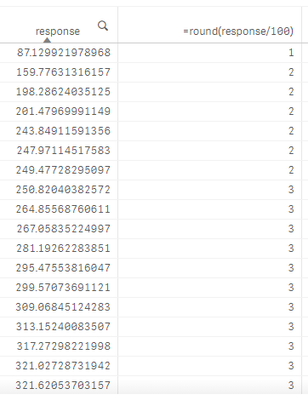# New to Qlik Sense

Discussion board where members can get started with Qlik Sense.

New Contributor

## Get counts of calculated meaure in a table

Hi,

I've made a table like this. I want to add a third column that contain counts of the second column (In this case, from top to bottom that would be: 1,6,6,6,6,6,6,11,11,11,11,11,11,11,11,11,11,11). What expression can I use to accomplish this?6 RepliesPartner

## Re: Get counts of calculated meaure in a table

I think count(distinct(round(response/100))) should work

Contributor III

## Re: Get counts of calculated meaure in a table

remove second expression as measure, create a new dimension(not measure) as round(response/100)

create a third measure as sum(aggr(count(1), round(response/100))

check it worksPartner

## Re: Get counts of calculated meaure in a table

If you could create a new dimension like:

Round((response)/100) as response_aggr

Your expression will be:

Count(total <response_aggr> response)

New Contributor

## Re: Get counts of calculated meaure in a table

This is along the lines of what I want, but it produces a column of all 1's

As for the other answers, I wish to do this all in a single measure if possible. In my actual app, the '100' is not fixed. It will be many different values for different chart objects so solving this with a new dimension in the data model is not a viable option.

Contributor III

## Re: Get counts of calculated meaure in a table

Did you check if adding this in measure sum(aggr(count(1), round(response/100)) works?  Just take one dimension I.e response.. I am guessing this should

New Contributor

## Re: Get counts of calculated meaure in a table

This produces a column of all 0s. I think this might be close though.